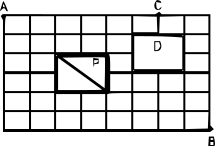# CAT 2008

Instructions

Directions for the next two questions: The figure below shows the plan of a town. The streets are at right angles to each other. A rectangular park (P) is situated inside the town with a diagonal road running through it. There is also a prohibited region (D) in the town.Question 11

Neelam rides her bicycle from her house at A to her office at B, taking the shortest path. Then the number of possible shortest paths that she can choose is

[CAT 2008]

Question 12

Neelam rides her bicycle from her house at A to her club at C, via B taking the shortest path. Then the number of possible shortest paths that she can choose is

[CAT 2008]

Instructions

For the following questions answer them individually

Question 13

Let $$f(x)\neq0$$ for any 'x' be a function satisfying $$f(x)f(y) = f(xy)$$ for all real x, y. If $$f(2) = 4$$, then what is the value of $$f(\frac{1}{2})$$?

Question 14

Suppose, the seed of any positive integer n is defined as follows:

seed(n) = n, if n < 10

seed(n) = seed(s(n)), otherwise, where s(n) indicates the sum of digits of n.

For example, seed(7) = 7,

seed(248) = seed(2 + 4 + 8) = seed(14) = seed (1 + 4) = seed (5) = 5 etc.

How many positive integers n, such that n < 500, will have seed (n) = 9?

Question 15

In a triangle ABC, the lengths of the sides AB and AC equal 17.5 cm and 9 cm respectively. Let D be a point on the line segment BC such that AD is perpendicular to BC. If AD = 3 cm, then what is the radius (in cm) of the circle circumscribing the triangle ABC?

Question 16

Consider obtuse-angled triangles with sides 8 cm, 15 cm and x cm. If x is an integer then how many such triangles exist?

Question 17

Consider a square ABCD with midpoints E, F, G, H of AB, BC, CD and DA respectively. Let L denote the line passing through F and H. Consider points P and Q, on L and inside ABCD such that the angles APD and BQC both equal 120°. What is the ratio of the area of ABQCDP to the remaining area inside ABCD?

Question 18

What is the number of distinct terms in the expansion of $$(a + b + c)^{20}$$?

Question 19

Five horses, Red, White, Grey, Black and Spotted participated in a race. As per the rules of the race, the persons betting on the winning horse get four times the bet amount and those betting on the horse that came in second get thrice the bet amount. Moreover, the bet amount is returned to those betting on the horse that came in third, and the rest lose the bet amount. Raju bets Rs. 3000, Rs. 2000 and Rs. 1000 on Red, White and Black horses respectively and ends up with no profit and no loss.

Which of the following cannot be true?

Question 20

Five horses, Red, White, Grey, Black and Spotted participated in a race. As per the rules of the race, the persons betting on the winning horse get four times the bet amount and those betting on the horse that came in second get thrice the bet amount. Moreover, the bet amount is returned to those betting on the horse that came in third, and the rest lose the bet amount. Raju bets Rs. 3000, Rs. 2000 and Rs. 1000 on Red, White and Black horses respectively and ends up with no profit and no loss.

Suppose, in addition, it is known that Grey came in fourth. Then which of the following cannot be true?

OR